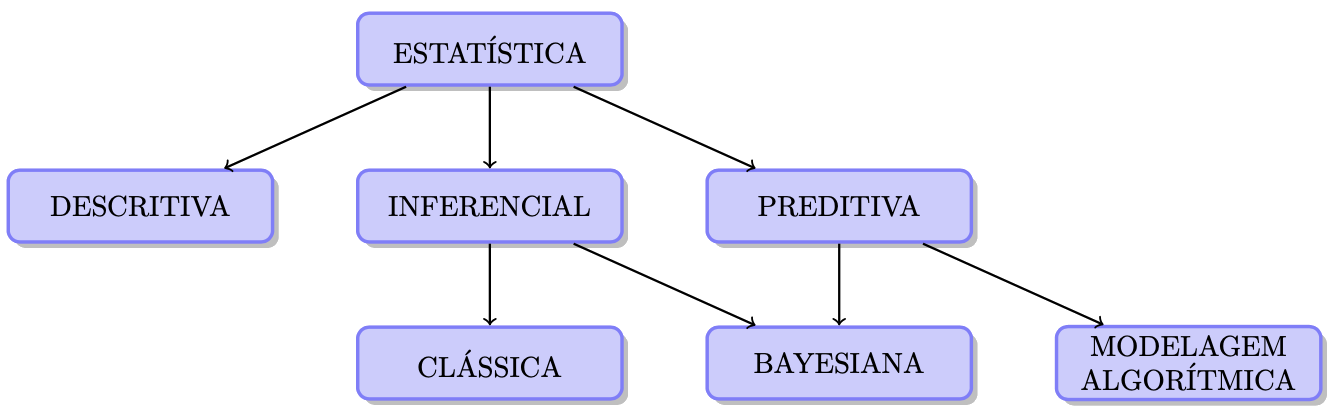# Chapter 1 Introduction

The object of statistical methods is the reduction of data.

The Statistical Method or simply Statistics brings together theoretical and practical tools to analyze quantitative information, measure uncertainties and help in decision making. It is a component of the Scientific Method, and can be divided according to the scheme in the Figure below. In this course, the fundamentals of topics such as exploratory data analysis, probability, sampling, inference under the classical and Bayesian prisms and linear models will be covered.A possible division of Statistics.

### References

Fisher, Ronald A. 1922. “On the Mathematical Foundations of Theoretical Statistics.” Philosophical Transactions of the Royal Society of London. Series A, Containing Papers of a Mathematical or Physical Character 222 (594-604): 309–68. https://royalsocietypublishing.org/doi/pdf/10.1098/rsta.1922.0009.